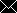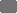# gms | German Medical Science

## GMS Medizinische Informatik, Biometrie und Epidemiologie

Deutsche Gesellschaft für Medizinische Informatik, Biometrie und Epidemiologie e.V. (GMDS)

ISSN 1860-9171

## Towards sequential statistical testing as some standard: Pearson’s correlation coefficient Hin zu sequentiellem statistischem Testen als Standardverfahren: Pearsons Korrelationskoeffizient

Research Article

### Suche in Medline nach

•Dieter Rasch - Department of Landscape, Spatial and Infrastructure Sciences, University of Natural Resources and Life Sciences, Vienna, Austria
•Takuya Yanagida - School of Applied Health and Social Sciences, University of Applied Sciences Upper Austria, Linz, Austria
•Klaus D. Kubinger - Division of Psychological Assessment and Applied Psychometrics, Faculty of Psychology, University of Vienna, Vienna, Austria
•Berthold Schneider - Institute for Biometry, Hannover Medical School, Hannover, Germany

GMS Med Inform Biom Epidemiol 2014;10(1):Doc07

Veröffentlicht: 28. Oktober 2014

Dieser Artikel ist ein Open Access-Artikel und steht unter den Creative Commons Lizenzbedingungen (http://creativecommons.org/licenses/by-nc-nd/3.0/deed.de). Er darf vervielfältigt, verbreitet und öffentlich zugänglich gemacht werden, vorausgesetzt dass Autor und Quelle genannt werden.

### Abstract

In standard statistical packages sequential tests are seldom and when they are offered, they mainly concern just tests about means. By a simulation study it was shown that a new sequential triangular test for the null-hypothesis H0: 0<ρρ0 for given requirements of precision (type-I-, type-II-risk, and a practical relevant effect δ=ρ1ρ0) offers reasonable results. Due to 100,000 runs the average sample size of the sequential triangular test is smaller than the sample size of the pertinent fixed sample size test. If practically possible, it is recommended that the triangular test should be used instead of a one-step procedure with a sample size fixed in advance.

Keywords: sequential test, correlation coefficient, simulation

### Zusammenfassung

In statistischen Programmpaketen findet man kaum sequentielle Tests und wenn doch, werden vor allem Erwartungswerte getestet. Mit Hilfe einer Simulationsstudie konnte gezeigt werden, dass ein neuer sequentieller Dreieckstest der Nullhypothese H0: 0<ρρ0 bei gegebenen Genauigkeitsforderungen wie Risiko erster und zweiter Art und einem praktisch relevanten Effekt δ=ρ1ρ0) zu vernünftigen Ergebnissen führt. Bei 100.000 simulierten Tests war der durchschnittliche Stichprobenufang des sequentiellen Dreieckstests kleiner als der entsprechende fest vorgegebene Umfang. Wenn es praktisch machbar ist, sollte stets der Dreieckstest an Stelle eines Tests mit fest vorgegebenem Umfang verwendet werden.

Schlüsselwörter: sequentieller Test, Korrelationskoeffizient, Simulation

### Introduction

In standard statistical packages sequential tests are seldom and when they are offered, they mainly concern just tests about means. For instance in , R-programs of sequential triangular tests can be found for:

• comparing a mean with a constant,
• comparing two means,
• comparing a probability with a constant,
• comparing two probabilities.

In this paper we present simulation results for a newly developed triangular sequential test for comparing a correlation coefficient with a constant.

In correlation analyses most of the time the null-hypotheses H0: ρ=0 (versus the two-sided alternative hypothesis HA: ρ≠0) is tested. But a significant correlation coefficient – regardless of how small the type-I-risk α may be established and the more regardless how small the p-value results – has often no practical meaning. Therefore it is often more reasonable to test the null-hypothesis H0: ρ=ρ0 for any 0<ρ0<1 against the alternative HA: ρ=ρ1 for any ρ1>ρ0 or ρ1<ρ0. Kubinger et al.  made available even to SPSS-users the fixed sample size test, which is known for this problem since a long time in statistics. In the present paper we show the attractiveness of a corresponding sequential triangular test proposed by Schneider et al. . In general, sequential triangular tests have the advantage that their average sample size is not only smaller than that of the corresponding fixed sample size tests but its maximum sample size needed is known in advance in any case.

### Method

The null-hypothesis H0: ρ=ρ0 can – without loss of generality – be replaced by H0: 0<ρρ0 or by H0: ρρ0. In an analogous way the alternative hypothesis may be replaced by a composite alternative.

Under the assumption that random variables are normally distributed with second moments σ2x, σ2y, and σxy, and a correlation coefficient ρ=σxy /(σxσy) the null-hypothesis should be tested against the alternative hypothesis HA: 0<ρ0ρρ1 with a type-I-risk α – i.e. the probability of wrongly rejecting H0 – and a type-II-risk β – i.e. the probability of wrongly accepting H0 (in particular as long as δρ1ρ0 with a ρ1 to be fixed in advance).

The empirical correlation coefficient r=sxy /sxsy based on k observations (xi,yi) (i=1,…,k) is an estimate of the parameter ρ (sxy, s2x, s2y are the empirical covariance and variances, respectively). In place of using r as a test statistic we use the transformed (Fisher transformation ) valueas a test statistic. The distribution of the corresponding random variable is approximately normal even if k is rather small. The expectation of z, being a function of ρ, amounts tothe variance toThe statistic z can be used to test for a fixed sample size k=n the hypothesis H0: ρρ0 against the alternative hypothesis HA: ρ>ρ0 (respectively H0: ρ≥ρ0 against H1: ρ<ρ0, a problem discussed in , Example 11.15). H0: ρρ0 is rejected with error probability α, if(respectively for H0: ρρ0, ifz1-α a is the (1-α)-quantile of the standard normal distribution).

An approximate lower bound for the sample size n which is necessary to keep a type-II-error with probability smaller or equal β given ρ=ρ1 is the smallest positive integer for which holds:.

A special group of sequential tests are the sequential triangular tests, going back to Whitehead  and Schneider . The main characteristic is that based on the actual sampled date, to decide whether sampling has to be continued or either the null- or the alternative hypothesis can be accepted.

We split the sequence of data pairs into sub-samples of length, say k>3 each. For each sub-sample j (j=1, 2,…, m) we calculate a statistic which distribution is only a function of ρ and k.

A triangular test must be based on a statistic with expectation 0, given the null-hypothesis, therefore we transform z into a realization of a standardized variable(1)
which has the expectation 0 if the null-hypothesis is true.

The parameter(2)
is used as a test-parameter. For ρ=ρ0 the parameter θ is 0 (as demanded). For ρ=ρ1 we obtain:(3)

The difference δ=ρ1ρ0 is the practical relevant difference which should be detected with the power 1–β.

From each sub-sample j we now calculate the sample correlation coefficient rj as well as its transformed values(j=1, 2,…, m).

Now byand Vm=m the sequential path is defined by points (Vm, Zm) for m=1,2,… up to the maximum of V below or exactly at the point where a decision can be made. The continuation region is a triangle whose three sides depend on α, β, and θ1 viaand(4),
with the percentiles zP of the standard normal distribution. That is, one side of the looked-for triangle lies between –a and a on the ordinate of the (V, Z) plane (V=0). The two other borderlines are defined by the lines L1: Z=a+cV and L2: Z=–a+3cV, which intersect at(5).

The maximum sample size is of course k·Vmax. The decision rule now is: Continue sampling as long as –a+3cVm<Zm<a+cVm if θ1>0 or –a+3cVm>Zm>a+cVm if θ1<0. Given θ1>0, accept HA in case that Zm reaches or exceeds L1 and accept H0 in case that Zm reaches or underruns L2, Given θ1<0, accept HA in the case Zm reaches or underruns L1 and accept H0 in the case Zm reaches or exceeds L2. If the pointis reached, HA is to be accepted.

### Example 1

We like to test the null hypothesis ρ≤.6 against the alternative hypothesis ρ>.6 with α=.05, β=.1 and δ=ρ1–.6 =.2. We use k=7. We hence obtainandSincewe obtainWe find z.0.9=1.282 and z.0.95=1.645, and henceFrom (5) we get Vmax=4.849/0.168=28.863, Zmax=9.698.

The corresponding triangle is shown in Figure 1 [Fig. 1].

### Example 2

Finally we demonstrate the case of Example 11.15 in . We like to test the null hypothesis ρ≥.8 against the alternative hypothesis ρ<.6 with α=.05, β=.1 and δ=.8–.6=.2. We use k=7. We hence obtainandSincewe obtainWe find z.0.9=1.282 and z.0.95=1.645, and henceFrom (5) we get Vmax=–4.849/–0.168=28.863, Zmax=–9.698.

The corresponding triangle is shown in Figure 2 [Fig. 2].

### Simulation study

The reasonability of the sequential triangular test for hypotheses of the correlation coefficient was tested by simulated paths (Z, V) being generated by bivariate normally distributed random numbers x and y with means µx=µy=0, variances σx2=σy2=1, and a correlation coefficient σxy=ρ. Simulations were performed with the nominal risks αnom=.05, βnom=.2 and several values of ρ0, ρ1 and k. For each parameter combination 100,000 paths were generated. Simulations as well as the calculation of the introduced sequential triangular test were done in R . As criteria for the test-quality we calculated: the relative frequency of wrongly accepting H1, given ρ=ρ0, which is the empirical risk of the first kind, say αemp; the relative frequency of rejecting H1, given ρ=ρ1 – this is empirical risk of the second kind, say βemp; the average number of samples ASN for ρ0 and ρ1 used for the calculation of r and z* until data sampling stopped (that is: the path leaves the continuation region). Here, ASN is the mean number of sample pairs over all 100,000 runs of the simulation study. Bear in mind that in a certain case the number of sample pairs needed for a terminal decision may lie either below or above that value ASN. The procedure is reasonable, if αempα and βempβ. Of course, the sequential test should also lead to ASN<nfix, where nfix is the sample size necessary in a fixed sample size test with a power 1–β, given ρ=ρ1 and testing the hypothesis ρρ0 with the type-I-risk α=.05. Results are shown in Table 1 [Tab. 1] where the difference between αemp and α are not to large. The ASN strongly depends on the real value of ρ. From Table 1 [Tab. 1] the reader finds reasonable values of k for the pairs (ρ0, ρ1) to be tested.

To summarize: If one actually wishes to check whether a correlation coefficient is large enough we suggest first to fix a lower bound of the coefficient of determination ρ02 as the value which at least must be given in order to speak of a meaningful percentage of the variance of y which can be explained by x – and vice versa. Another value of the coefficient of determination, ρ12, is that one which the researcher must not oversee with a higher probability than 1–β if given. It is easy to adapt the procedure for negative roots of these squares. For example, one might decide for ρ02=.5, as a consequence of which ρ0=.7; suppose ρ1=.8, α=.05, β=.2, then using k=26 (by interpolation) a total sample size will result as about 108 instead of 119 for a fixed sample study.

### Note

#### Conflict of interest

The authors declare that they have no competing interests.

### References

1.
Rasch D, Pilz J, Verdooren RL, Gebhardt A. Optimal experimental design with R. New York: Chapman & Hall/CRC; 2011.
2.
Kubinger KD, Rasch D, Šimeckova M. Testing a correlation coefficient's significance: Using H0: 0<ρ≤λ is preferable to H0: ρ=0. Psychol Sci. 2007;49:74-87.
3.
Schneider B, Rasch D, Kubinger KD, Yanagida T. A Sequential Triangular Test of a Correlation Coefficient's Null-Hypothesis: 0<ρ≤ρ0. Stat Pap. 2014 Jun 12. DOI: 10.1007/s00362-014-0604-84.
Fisher RA. Frequency distribution of the values of the correlation coefficient in samples from an indefinitely large population. Biometrika. 1915;10(4):507-21.
5.
Rasch D, Kubinger KD, Yanagida T. Statistics in Psychology. Using R and SPSS. Chichester: Wiley; 2011. DOI: 10.1002/97811199796306.
Whitehead J. The Design and Analysis of Sequential Clinical Trials. 2nd ed. Chichester: Ellis Horwood; 1992.
7.
Schneider B. An interactive computer program for design and monitoring of sequential clinical trials. In: Proceedings of the XVIth international biometric conference; 1992 Dec 7-11; Hamilton, New Zealand. p. 237-50.
8.
R Core Team. R: A language and environment for statistical computing. Vienna, Austria: R Foundation for Statistical Computing; 2013. Available from: http://www.R-project.org/